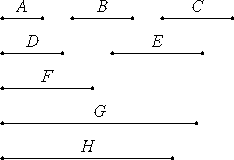# Proposition 39

To find the number which is the least that has given parts.

Let A, B, and C be the given parts.

It is required to find the number which is the least that will have the parts A, B, and C.VII.36

Let D, E, and F be numbers called by the same name as the parts A, B, and C. Take G, the least number measured by D, E, and F.

VII.37

Therefore G has parts called by the same name as D, E, and F.

But A, B, and C are parts called by the same name as D, E, and F, therefore G has the parts A, B, and C.

I say next that it is also the least number that has.

If not, there is some number H less than G which has the parts A, B, and C.

VII.38

Since H has the parts A, B, and C, therefore H is measured by numbers called by the same name as the parts A, B, and C. But D, E, and F are numbers called by the same name as the parts A, B, and C, therefore H is measured by D, E, and F.

And it is less than G, which is impossible. Therefore there is no number less than G that has the parts A, B, and C.

Q.E.D.

## Guide

The wording of the proposition is somewhat unclear, but an example will show its intent.

Suppose you want to find the smallest number with given parts, say, a fourth part and a sixth part. Then take the LCM(4,6) which is 12. The number 12 has a 1/4 part, namely 3, and a 1/6 part, namely 2.